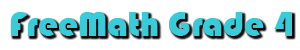Begin 1st Nine Weeks Place Value 1A Thousands: value of underlined 1B Thousands: read and write numbers 1C Hundred Thousands: value of underlined 1D Hundred Thousands: word form & expanded 1E Millions: value of underlined digit 1F Millions: read and write numbers 1G Estimating: benchmark numbers Compare and Order Numbers 1H Compare Numbers 1I Order Numbers: least to greatest Order Numbers: greatest to least Money 2A Write amount shown 2B Problem Solving: making change 2C Compare Money 2D Order Money: least to greatest 2E Order Money: greatest to least Rounding 2F Round Numbers: nearest ten thousand 2G Round Numbers: nearest hundred thousand 2H Round Numbers: nearest million 2I Estimate Sums & Differences - thousand 2J Estimate Sums & Differences - ten thousand 2K Problem Solving: rounding numbers Addition and Subtraction 3A Addition: four-digit numbers 3B Subtraction: four-digit numbers 3C Subtract Across Zeros Algebra 3D Expressions (no variable) 3E Expression (with variable) 3F Addition Properties 3G Equations 3H Related Equations 3I Problem Solving: addition four-digit Time 4A Circle Time on Clock 4B Elapsed Time Collect and Organize Data 4C Tally Table 4D Frequency Table 4E Median 4F Mode 4G Line Plot 4H Organize Data - Stem & Leaf Plot 4I Problem Solving: Time Graphs 5A Bar Graph 5B Line Graph 5C Horizontal Bar Graphs 5D Circle Graphs 5E Bar Graphs from Tables 5F Bar Graphs from Tables 5G Line Graphs 5H Making Circle Graphs Multiplication and Division 6A Fact Families: related division fact 6B Fact Families: complete the family 6C Fact Families: write the family 6D Multiplication Properties 6E Expressions with Variables Equations 6F Problem Solving: Equations w/Variables 6G Balance Equations Multiplication 7A Multiply by One-Digit 7B Multiply by One-Digit with Regrouping 7C Square Numbers Number Theory 7D Square Numbers 7E Factors 7F Multiples 7G Prime and Composite Numbers 7H Problem Solving: Multiplication Division 8A Dividing Two-Digit Numbers 8B Vocabulary 8C Missing Factors 8D Expressions 8E Division Word Problems Multiplication 9A Multiply by One-Digit Numbers 9B Multiply by One Digit w/Regrouping 9C Rounding 9D Estimate Products 9E Problem Solving: Multiplication Graphing Functions 10A Use Coordinate Graph 10B Equation to Complete Table 10C Equation that Shows Data 10D Graph that Shows Equation 10E Problem Solving Multiplication 11A Multiply with Zeros 11B Multiply by 6 and 7 11C Multiply by 8 and 9 11D Multiply Two-Digit by Two-Digit 11E Problem Solving 12A Estimate Products 12B Multiply Three-Digit by Two-Digit 12C Multiply Tens and Hundreds 12D Problem Solving Division 13A Dividing Three-Digit Numbers 13B Dividing with Remainders 13C Dividing Three-Digit Numbers by 6 and 7 13D Dividing by 8 and    9 13E Estimate Quotients 13F Zeros in Quotients 13G Divisibility 13H Problem Solving 14A Divide Five-Digit Numbers 14B Find the Mean 14C Problem Solving 15A Two-Digit Divisors 15B Dividing by Tens 15C Problem Solving Multiplication and Division 16A Order of Operations 16B Negative Numbers 16C Inequalities Measurement: Customary Units 17A Choose Unit of Measure 17B Fractional Measures 17C Equivalent Measures 17D Capacity: Equivalent Measure 17E Capacity: Equivalent Measure Measurement: Metric Units 18A Length: Centimeter 18B Length: Equivalent Measure 18C Length: Equivalent Measure 18D Mass Customary and Metric Units 18E Problem Solving Solid Figures 19A Faces, Edges, Vertices Plane Figures 19B Polygons 19C Polygons 19D Classify Triangles 19E Classify Quadrilaterals 19F Circles Geometry 19G Points, Lines, Segments, Rays 19H Classify Angles 19I Classify Lines 20A Symmetry 20B Congruent & Similar Figures 20C Transformations 20D Tessellations 20E Perimeter 20F Circumference 20G Area 20H Volume Fractions 21A Read and Write Fractions 21B Equivalent Fractions 21C Simplest Form 21D Compare Fractions 21E Order Fractions: least to greatest 21F Order Fractions: greatest to least 21G Mixed Numbers 21H Mixed Numbers Probability 22A Outcomes: Spinners 22B Outcomes: Marble Bag 22C Outcomes: Spinners 22D Problem Solving: Combinations Add Fractions 23A Add Like Fractions 23B Add Like Fractions: find x 23C Add Mixed Numbers 23D Add Unlike Fractions Subtract Fractions 24A Subtract Like Fractions 24B Subtract Like Fractions: find x 24C Subtract Mixed Numbers 24D Add & Subtract Mixed Numbers 24E Subtract Unlike Fractions Decimals 25A Tenths 25B Hundredths 25C Thousandths 25D Fraction as Decimal: tenths, hundredths 26A Compare Decimals & Fractions 26B Estimate Sums & Differences 27A Round Decimals 27B Estimate Sums & Differences 27C Add Decimals 27D Subtract Decimals 27E Problem Solving